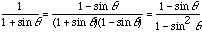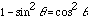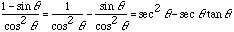Date: Sat, 27 Jun 1998 19:37:06 -0700
Subject: A calculus problem

I'm a post-secondary student taking calculus by correspondence. I'm stuck on the following question (and similar ones) Can you help?

Evaluate the following indefinite integral:

d(theta)
----------
1 + sin (theta)

(It says to multiply both numerator and denominator by: 1 - sin(theta)

Thanks
Lorraine

Hi Lorraine

If you multiply the numerator and denominator as suggested the integrand becomesButso the integrand can be written asand this is an integrand you should be able to integrate.

Cheers
Harley

Go to Math Central